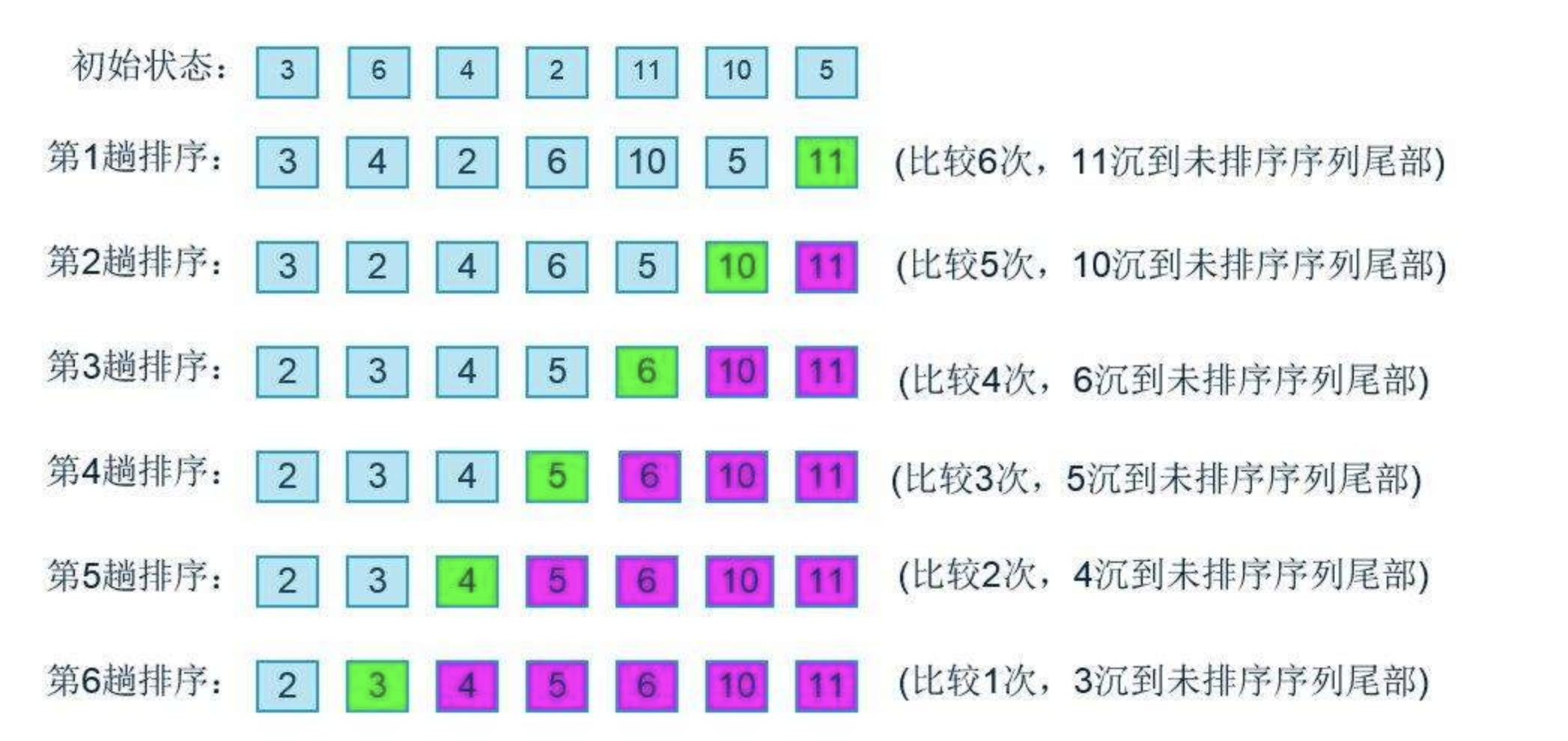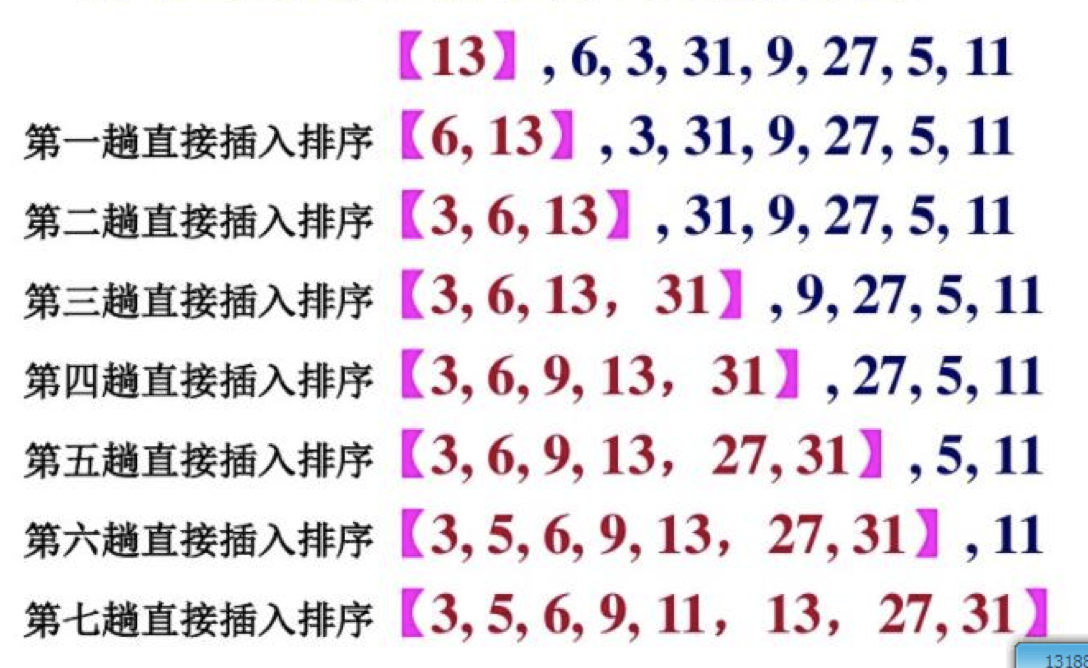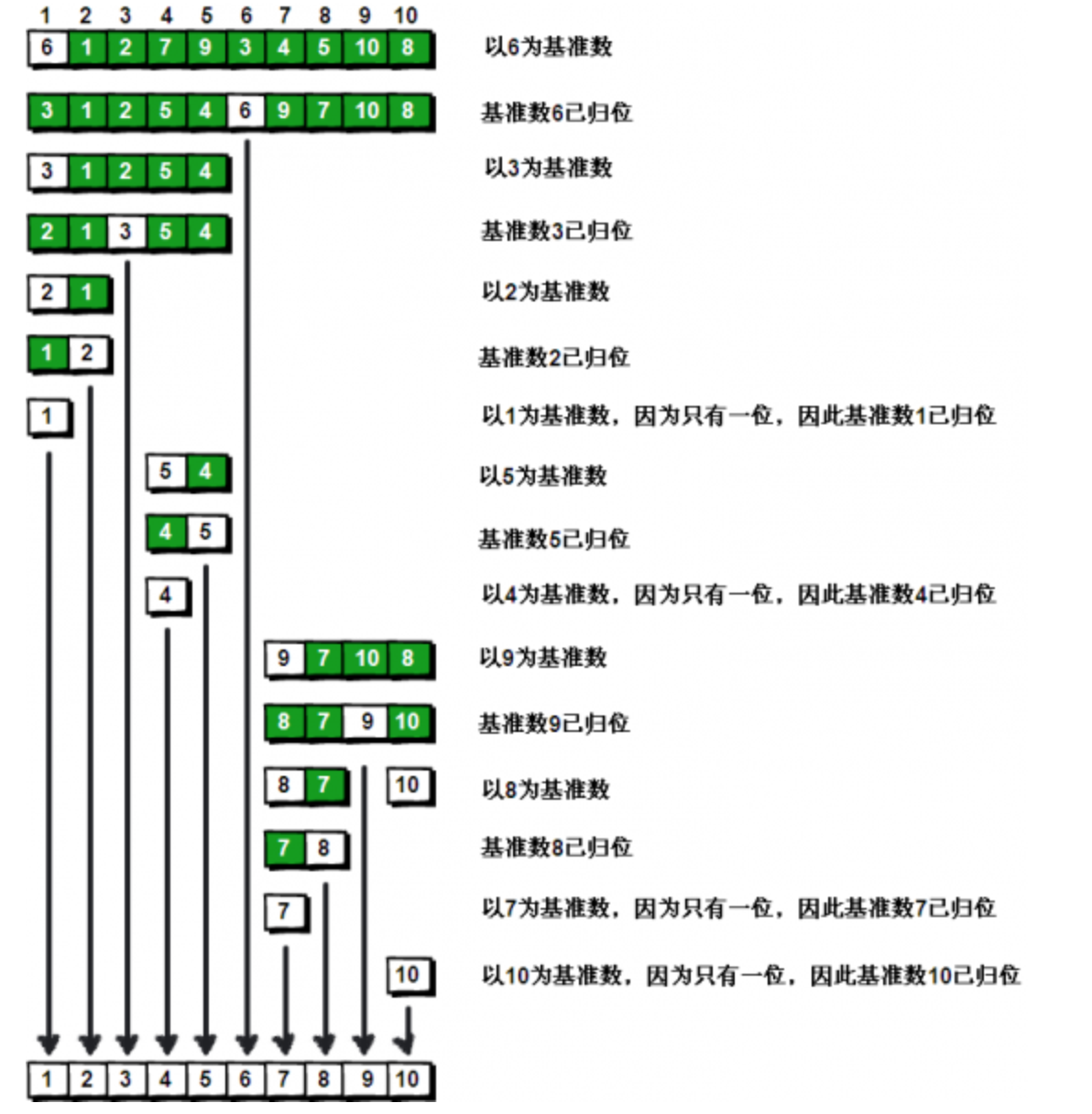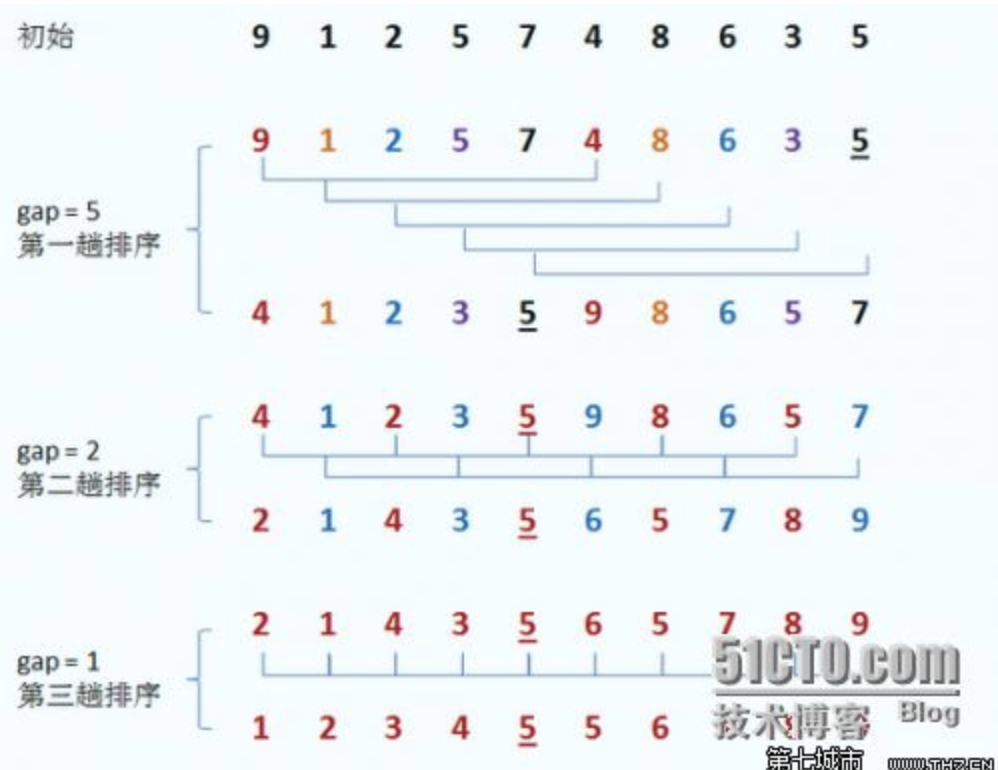# 1、排序算法

## 1.1、冒泡排序（O(n^2)）@Test
public void test(){
int nums[] = {49, 38, 65, 97, 76, 13, 27, 50};
冒泡排序(nums);
System.out.println(Arrays.toString(nums));
}

public void 冒泡排序(int[] nums) {
// i=> 排序次数（最多做n-1趟排序）
for (int i = 1; i < nums.length; i++) {
//j，当前位置指针 j最大不能超过 str.length - i
for (int j = 0; j < nums.length - i; j++) {
if (nums[j] > nums[j + 1]) {
int temp = nums[i];
nums[i] = nums[j];
nums[j] = temp;
}
}
}
}


### 1.1.1、冒泡排序优化

public void 冒泡排序优化(int[] nums) {
// i=> 排序次数（最多做n-1趟排序）
for (int i = 1; i < nums.length; i++) {
//是否发生交换
boolean flag = false;
//j，当前位置指针 j最大不能超过 str.length - i
for (int j = 0; j < nums.length - i; j++) {
if (nums[j] > nums[j + 1]) {
flag = true;
int temp = nums[i];
nums[i] = nums[j];
nums[j] = temp;
}
}

//当一趟比较没有发送交换的时间表示已经有序
if (!flag) {
break;
}
}
}


## 1.2、选择排序

1、首先在未排序序列中找到最小（大）元素，存放到排序序列的起始位置。

2、 再从剩余未排序元素中继续寻找最小（大）元素，然后放到已排序序列的末尾。

3、重复第二步，直到所有元素均排序完毕。

1、时间复杂度：(n^2)

@Test
public void test(){
int[] nums = {49, 38, 65, 97, 76, 13, 27, 50};
选择排序(nums);
System.out.println(Arrays.toString(nums));
}

public void 选择排序(int[] nums) {
//从前往后比较，从0开始，是因为它要赋值给min,i一直到a.length 也就是最后一个还需要往前移动
for (int i = 0; i < nums.length - 1; i++) {
//首先默认第一个为最小值
int idx = i;
//是和tId比较 ，不需要自己跟自己比较，min初始给的i，所以j=i+1;
for (int j = i + 1; j < nums.length; j++) {
if (nums[idx] > nums[j]) {
idx = j;
}
}

//每趟排序之后，idex的值都会不一样 ,而每次的min都是开始的i，所以当下的i和min进行替换
if (idx != i) {
int temp = nums[i];
nums[i] = nums[idx];
nums[idx] = temp;
}
}
}


## 1.3、直接插入排序(O(n^2))

1、时间复杂度O(n^2)：

当问题规模为n时



2、空间复杂度：

3、算法稳定性：1、直接插入排序 ：从第二个开始，依次和前一个进行比较，插入一个有序序列（注意和选择排序的区别）


/**
* 1、直接插入排序 ：个人理解，就是往后移动，依次把小的放到前面来
*/
@Test
public  void insertionSort() {
int[] a = { 49, 38, 65, 97, 76, 13, 27, 50 };
System.out.println("----------插入排序开始：---------");
print(a);
for (int i = 1; i < a.length; i++) {//从i等于1开始表示a 也即是从第二个数字开始进行比较，进行n-1趟排序
// 待插入元素
int temp = a[i];
int j ;
for (j = i; j >  0; j--)
{
// 将大于temp的往后移动一位,其实就是和temp进行比较移动，已经排序的二舅不会移动了
if (a[j-1] > temp)
{
a[j] = a[j-1]; //执行完这个 j之后还要 继续执行下一个  j 最后代表的就是 实际 带待插入元素的位置
}
else
{
break;
}
}

a[j] = temp; //，如果不变则原封不动给它(主要原因)，如果变了则将它赋值给j  进行归为，此时的j就是我们上面排序之后找到的j的位置

System.out.printf("第"+i+"趟排序结果,");
print(a);
}

System.out.print("最终插入排序结果： ");
print(a);
System.out.println("--------------------");
}

/**
*
打印的结果
*/
private static void print(int []a) {
for (int i : a){
System.out.print(i + " ");
}
System.out.println();
}



## 1.4、快速排序(O(log2^n))

1．先从数列中取出一个数作为基准数。下面

2．分区过程，将比这个数大的数全放到它的右边，小于或等于它的数全放到它的左边。

3．再对左右区间重复第二步，直到各区间只有一个数。@Test
public void test() {
int nums[] = {49, 38, 65, 97, 76, 13, 27, 50};
快速排序(nums);
System.out.println(Arrays.toString(nums));
}

public void 快速排序(int[] nums) {
int low = 0;
int high = nums.length - 1;
sort(nums, low, high);
}

public void sort(int[] nums, int low, int high) {
int i = low, j = high;

//从左右两边交替扫描，直到left = right
if (i < j) {
//待排序的第一个元素作为基准值
int po = nums[low];

//每次当i比j小的时候小的时候开始比较，当它大于的时候，就会开始下一次排序
while (i < j) {

//从右往左扫描，找到第一个比基准值小的元素
while (i < j && po < nums[j]) {
j--;
}

//找到这种元素将nums[j]放入nums[i]中
nums[i] = nums[j];

//从左往右扫描，找到第一个比基准值大的元素
while (i < j && po > nums[i]) {
i++;
}

//找到这种元素将nums[i]放入nums[i]中
nums[j] = nums[i];
}

//基准值归位 这个时候 (这里i和j是相等的)
nums[i] = po;

//对基准值左边的元素进行递归排序(这里i和j是相等的)
sort(nums, low, i - 1);

//对基准值右边的元素进行递归排序。
sort(nums, i + 1, high);
}
}



## 1.5、希尔排序(n^1.3)@Test
public void test() {
int nums[] = {49, 38, 65, 97, 76, 13, 27, 50};
希尔排序(nums);
System.out.println(Arrays.toString(nums));
}
public void 希尔排序(int nums[]) {
//希尔排序增量，//被分成4组 ，也即是第1个和第5个进行比较 ，低2个和低6个比较
int incr = nums.length / 2;
//当增量为0的时候排序完成
while (incr > 0) {
//以为是从前往后第一个数字开始比较，所以初始化i=0 ，插入排序是从后往前比较, 小于a.length 表示的是有坑呢到最后分成最后一组的时候会 相互挨着的笔记，所以一定要到结尾
for (int i = 0; i < nums.length - 1; i++) {
// 这里的每一趟相当于是一次插入排序的排序算法，不同的是，这里是从前往后
for (int j = i; j < nums.length - incr; j = j + incr) {
if (nums[j] > nums[j + incr]) {
int temp = nums[j + incr];
nums[j + incr] = nums[j];
nums[j] = temp;
}
}
}
incr = incr / 2;
}
}


# 2、时间复杂度求法

https://www.cnblogs.com/dragondove/p/6389177.html

## 2.1、时间复杂度

 时间复杂度可以认为是对排序数据的总的操作次数。反映当n变化时，操作次数呈现什么规律。

常见的时间复杂度有：常数阶O(1),对数阶O(log2n),线性阶O(n), 线性对数阶O(nlog2n),平方阶O(n2)

时间复杂度O(1)：算法中语句执行次数为一个常数，则时间复杂度为O(1),


## 2.2、空间复杂度

空间复杂度是指算法在计算机内执行时所需存储空间的度量，它也是问题规模n的函数

ax=N，则x=logaN，



## 2.3、排序时间复杂度的记忆法则

快速最烦人



# 3、折半查找，二分查找O(log2n)

/**
* @Description
* @Author HealerJean
* @Date 2018/4/24  下午12:09.
*/
public class 折半查找 { //先有序，再折半查找
public static void main(String[] args) {

int array[]=new int[]{1,2,3,4,5,6,7,8,9,10,11,12,13,14,15,16,16,17,18,19,20};
int low=0;
int high=array.length-1;
int mid;
int x=20;
while(low<=high){ //最后的情况一定是相等
mid=(low+high)/2;
if(array[mid]==x){
System.out.println(x+"在数组中出现的位置"+mid);
break;
}
if(array[mid]<x){
low=mid+1;
}
if(array[mid]>x){
high=mid-1;
}
if(low>high){
System.out.println("查找失败");
break;
}
}

}

}